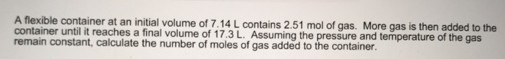# Problem: A flexible container at an initial volume of 7.14 L contains 2.51 mol of gas. More gas is then added to the container until it reaches a final volume of 17.3 L. Assuming the pressure and temperature of the gas remain constant, calculate the number of moles of gas added to the container.

###### FREE Expert Solution
97% (90 ratings)###### Problem Details

A flexible container at an initial volume of 7.14 L contains 2.51 mol of gas. More gas is then added to the container until it reaches a final volume of 17.3 L. Assuming the pressure and temperature of the gas remain constant, calculate the number of moles of gas added to the container.